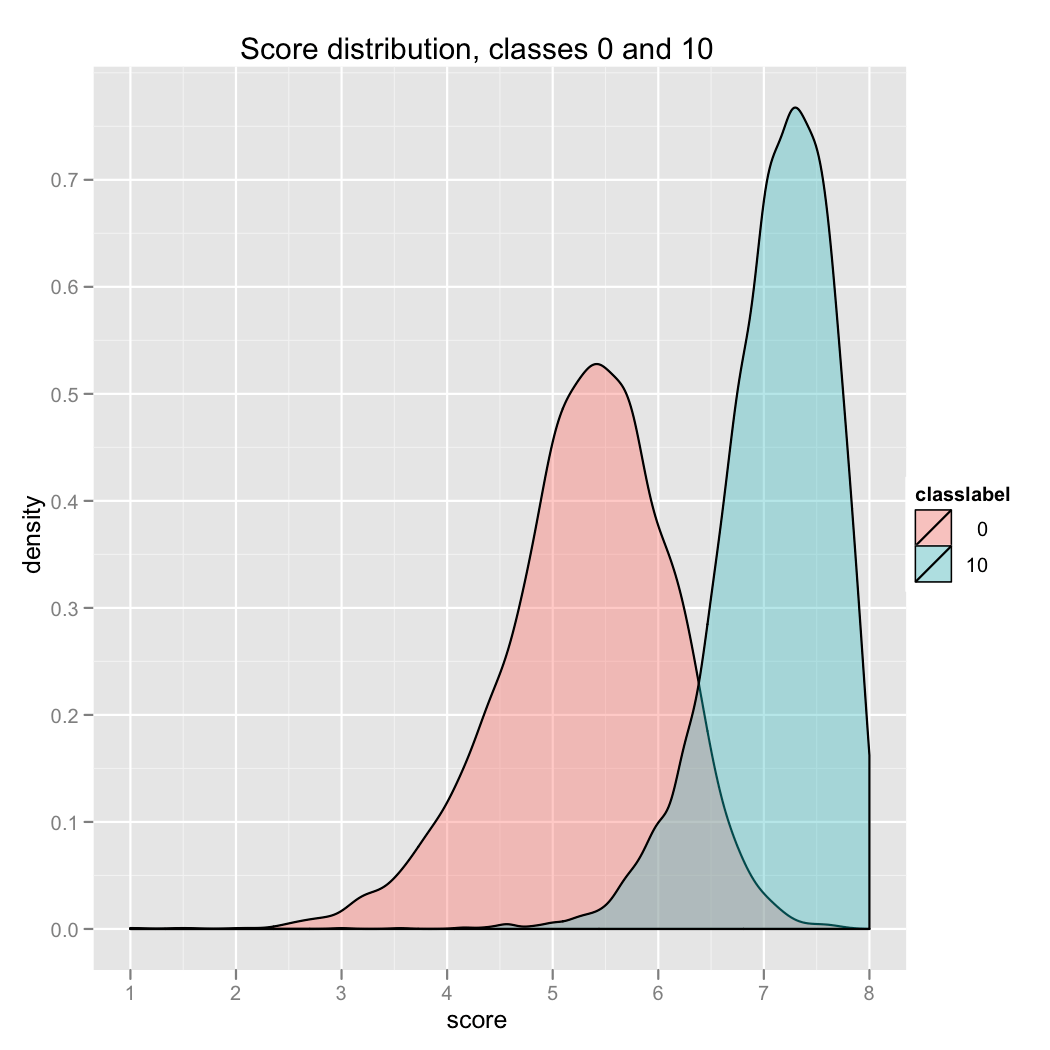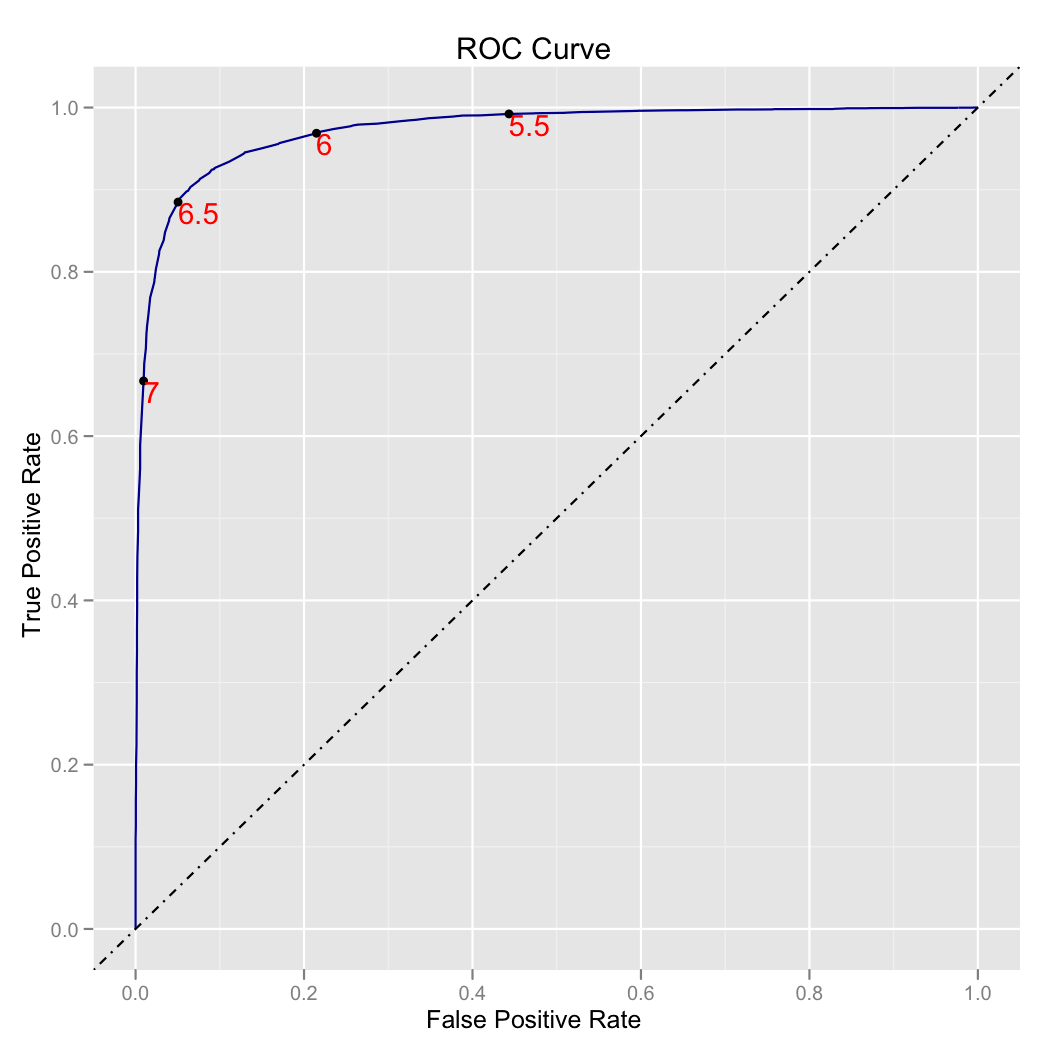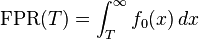# More on ROC/AUC

A bit more on the ROC/AUC

## The issue

The receiver operating characteristic curve (or ROC) is one of the standard methods to evaluate a scoring system. Nina Zumel has described its application, but I would like to call out some additional details. In my opinion while the ROC is a useful tool, the “area under the curve” (AUC) summary often read off it is not as intuitive and interpretable as one would hope or some writers assert.

The situation where a ROC analysis is useful is quite common. Typically it is in a machine learning situation where you are training a classifier to decide between two categories. We will call the categories “true” and “false”. Many machine learning techniques (regression, Naive Bayes, logistic regression, SVM and so on) actually produce something more useful than a single classifier. They produce a scoring function where, if the classifier is working well, higher score indicates a higher probability of an item belonging to the “true” class. To turn a scoring function into a classifier (or decision procedure) all that is needed is a cut-off score (called a threshold). All items receiving a score above the threshold are called “positive” (hopefully the predicted analogue for “true”) and all items receiving a score below the threshold are called “negative” (hopefully the predicted analogue for “false”). By picking different thresholds one can get different (though related classifiers) that represent different trade-offs between true_positive_rate (also called sensitivity) and false_positive_rate (also called fall-out, see ROC). Or (equivalently) we are only concerned with trade-offs among competing classifier performance metrics such as precision, recall and specificity. To our mind this is best graphical representation of the the family of classifiers derivable from a single scoring function is the “double hump graph.” This is either a pair of density plots (where areas are re-normalized to integrate to one) or pair of histograms (where area is proportional to number of training examples) that simultaneously show the score distribution on true and false examples. If the two distributions are somewhat separated we get a family of thresholds that yield high quality classifiers. If the two distributions are identical we have nothing.

An example of a double-hump graph (from “I don’t think that means what you think it means;” Statistics to English Translation, Part 1: Accuracy Measures) is given here:From the same source the ROC curve is as follows:The ROC curve’s strength and weakness is the observation that classifier score is itself a nuisance parameter. Beyond the effects on classifier behavior we do not care about actual values of the score. We only care about the different true_positive_rates and false_positive_rates that are simultaneously achievable, not what scores achieve them (that is in implementation detail). The ROC plot is exactly the plot of the efficient frontier ofsimultaneous true_positive_rates and false_positives_rates. We will abbreviate these rates as tpr and fpr. The ROC plot can be produced by for every possible threshold: compute the tpr and fpr induced by this threshold and add the point (tpr,fpr) to the curve. Notice: the threshold does not make it to the graph- it is lost in in the parametric description of the ROC curve. What is missing is: the speed the score parameter is moving along the curve and what density of data is associate with each score. These is evident in the double hump plots where they are drawn as areas.

An issue is interpreting the ROC graph. I remember seeing a presenter show a classifier they were proud of at KDD where the curve nearly followed the diagonal line y=x. The presenter appeared blissfully unaware that random selection achieves this useless level of performance. However, it is natural to talk about area under the curve (AUC), it is what you see so you should have some feel for it. The first thing to remember: is you get the first 1/2 units of area for free (no credit to you there) it is only the second 1/2 that is at all meaningful. One should be suspicious area on ROC graphs. Typically you are going to pick only one threshold and live with that classifier, the perceived performance of classifiers you are not using is not entirely relevant. Also many scoring functions, for example those coming from naive Bayes are in fact highly concentrated and really only yield one usable classifier.

There is an extreme desire to bind AUC quickly to intuition.

## One interpretation

For example, “Why do Nigerian Scammers Say They are from Nigeria?” Cormac Herley states: “The Area Under the Curve (AUC) is the probability that the classifier ranks a randomly chosen true positive higher than a randomly chosen false positive.” This actually can not be true just on the face of it. Which examples (and at what rate) are positive and negative (let alone true positive and false positive) is a function of what single threshold is chosen. So one of the two statements (comparison rates of true positives to false positives) varies with threshold and the other (AUC) does not (as it is a graph over all thresholds). The two quantities can not, in general, be the same.

That is perhaps a mangling of Wikipedia’s: “The area under curve (AUC), when using normalized units, is equal to the probability that a classifier will rank a randomly chosen positive instance higher than a randomly chosen negative one (assuming ‘positive’ ranks higher than ‘negative’).” We are assuming they are using positive/negative as we are using true/false (and will make the necessary translations). Which the Wikipedia in turn attributes to Fawcett, Tom (2006) “An introduction to ROC analysis”, Pattern Recognition Letters, 27, 861–874. The paper is behind the usual inexcusable ACM paywall, so we are not going to go to the original reference to confirm the source of this misleading statement. So I can not, of course, presume to criticize the calculations and lessons of “An introduction to ROC analysis”, but I can criticize the implication that area has an obvious (one not needing to be tutored in) interpretation.

There are corner-case examples (involving duplicate data) that violate the claim (unless you add sufficient tie-breaking or integrate over score perturbation rules). Consider the following trivial data set:

datum number classifier score actual class
1 0.1 false
2 0.1 false
3 0.1 true
4 0.9 true

The only interesting score thresholds are score≥0.1 and score≥0.9. We know fpr = true_positives/total_positives and fpr = false_positives/total_negatives. So:

• At score≥0.1 we have tpr = 2/2 and fpr = 2/2
• At score≥0.9 we have tpr = 1/2 and fpr = 0/2

So the AUC is 3/4. But the probability of (a random true example scores above random false example) is: 1/2 as all false examples have score 0.1 and half the true examples have score>1/2. The two numbers do not agree when we compute expectations with respect to the training data (the natural or empirical way to estimate this value).

### The continuous argument

Note: in early drafts of this I thought the corner-case counter-example obvioulsy ruined the interpretation in all cases, I was wrong.

The argument given in the Wikipedia involves integrating across all classifier thresholds the following:

Where `f0()` and `f1()` are defined so thatNotice the argument’s use of integrals as inverse derivatives (the fundamental theorem of calculus) which we will call “the continuous case.” Statistics often uses more sophisticated integrals (such as Lebesgue) which can deal with discrete jumps, but not all the steps of the argument hold in that case (as seen in our above corner case counter example).

In the continuous case we can interpret the first step as calculating area by integrating `TPR(inverse-FPR(x))` under a change of variables `x = FPR(T)`. The integral also has an interpretation as the expected true positive rate sampled proportional to changes in false positive rate, as we expect `integral_{T=-inf,+inf} (FPR'(T)dT) = 1`. In our case (where FPR(T) only changes int two places) we must have `A = lambda (1/2) + (1-lambda) (1)` for some lambda in [0,1] (lambda plausibly =1/2). So we do get the expected 3/4- but this is not the probability a random uniformly chosen positive example scores higher than a uniformly chosen negative example (which is “1/2” for strictly greater, and “1” for greater than or equal). However obvious tie-breaking rules would let us say the “tie-broken probability of a true item scoring higher than a false item” agrees with the area.

One might wonder why we care about discrete data with ties? The reason is this is what we will encounter in the real world, the interpretation of `TPR` and `FPR` as integrals of simple continuous (non-atomic) distributions is, while convenient, a bit unnatural. In fact the distributions we see in the real world are “atomic” (have non negligible mass at specific points), so it is a bit disingenuous to use a smooth argument style on them (basic Riemann style integrals) without checking the consequences. In fact the types of integral theories needed to work with the actual types of distributions you see from finite data (Lebesgue integrals or operator theories of distributions) are a bit more subtle (don’t always obey the fundamental theorem of calculus in that integrals are not always anti-derivatives) and for this application introduce a counting of 1/2 for ties (not an unreasonable thing, but not the stated claim!).

I myself got this wrong in criticizing “uniformity” assumptions in the early (incorrect) version of this note, when the real issue was atomic measures (measures with jumps in their cumulative distributions). But the issue remains: the re-shared statements are overly simplified to the point where they are not technically correct.

### The correct discrete argument

The continuous argument can, quickly be adapted to the discrete case.

Without loss of generality assume our scores are exactly the integers 1 through k.

Define:

• `f1(i) = P[score = i | item is true ]`
• `f0(i) = P[score = i | item is false ]`
• `TPR(j) = sum_{i=j...k} f1(i)`
• `FPR(j) = sum_{i=j...k} f0(i)`

Use the trapezoid rule to compute area A:

``` A = Sum_{i=1...k} (1/2)(TPR(i)+TPR(i+1)) * (FPR(i)-FPR(i+1)) = Sum_{i=1...k} ( (1/2) f1(i) + TPR(i+1) ) * f0(i) = Sum_{i=1...k} TPR(i+1) * f0(i) + (1/2) f1(i) * f0(i) ```

Which (after following the pattern of the original continuous argument a bit further) fits the rule of: 1 point every time a true example scores above a negative example, and 1/2 point when they have the same score. Not the rule stated in the references (so they also had an issue), but also not an unnatural counting rule.

## Conclusion

The ROC curve is a useful tool, but you have to use it for appropriate tasks. We know when looking at it you can’t help but directly see the area (AUC). However, in our opinion, the AUC does not have as straightforward a business intuition as we would hope. Indeed, over actual data usually only a few high-quality classifiers can be built by choosing threshold (due to the score often being concentrated at a few values). This contradicts the expectation formed from the diagram that you have easy access to many classifiers with a wide range of dial-able behaviors. Without an explicit cost of error model (cost of false positives and separate cost of false negatives) you should always be suspicious of a single number summary of a classifier performance (be it accuracy, AUC, F1 or so on). We in fact prefer using both precision and recall. If you insist on a single number: the F1 is a good heuristic measure of classifier quality, as it at least incorporates our operational choice of score threshold into the quality assessment. The ROC curve is useful tool designing a classifier from a scoring function (though I prefer the “double hump graph”), but once you have chosen a threshold the performance of the other classifiers (induced by choosing different thresholds) are irrelevant to assessing the performance of the classifier you have settled on.

Categories: Opinion Statistics Tutorials### jmount

Data Scientist and trainer at Win Vector LLC. One of the authors of Practical Data Science with R.

### 2 replies ›

1.jmount says:

@zubin
Thanks, I like the reference. I think the misinterpretations I criticize may be related to the page 106 Whitney-Wilcoxon example you give (which, while correct in no way sounds useful- I suspect the author didn’t like it either). And the line “yet another interpretation of AUC is the average sensitivity, regarding values of the specificity as equally likely” is pretty much my complaint (as regarding specificity as being uniformly distributed is usually not consistent with the training data).